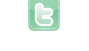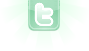This site requires JavaScript, please enable it in your browser!please can some one help me with this code

so basically i want an actor call "blueCircle" to move in 1 direction at a random and move at the same speed the whole time it also needs to move in a straight line, please help, thank you
To consistently move the same speed and in a straight line in any direction, use a smooth moving system.
please could you give me an example of this
Coltz wrote...
please could you give me an example of this
public class Bullet extends Actor
{
double x, y, dx, dy;
int speed = 5;

protected void addedToWorld(World world)
{
x = getX();
y = getY();
dx = Math.cos(Math.PI*getRotation()/180)*speed;
dy = Math.sin(Math.PI*getRotation()/180)*speed;
}

public void act()
{
move();
}

public void move()
{
x += dx;
y += dy;
setLocation((int)x, (int)y);
}
}
In the above example, the rotation is set before the bullet is added into the world.
You need to login to post a reply.